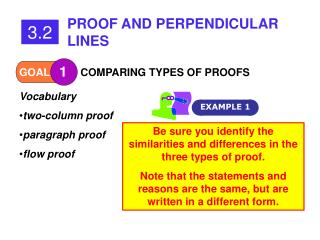DownloadDownload PresentationGOAL

# GOAL

Download Presentation## GOAL

- - - - - - - - - - - - - - - - - - - - - - - - - - - E N D - - - - - - - - - - - - - - - - - - - - - - - - - - -
##### Presentation Transcript

1. PROOF AND PERPENDICULAR LINES 3.2 COMPARING TYPES OF PROOFS 1 GOAL EXAMPLE 1 • Vocabulary • two-column proof • paragraph proof • flow proof Be sure you identify the similarities and differences in the three types of proof. Note that the statements and reasons are the same, but are written in a different form.

2. A B C D Extra Example 1 Given: AB = CD Prove: AC = BD StatementsReasons 1. AB = CD 1. Given 2. 2. 3. 3. 4. 4. Addition prop. of = AB + BC = BC + CD AB + BC = AC and BC + CD = BD Segment Addition post. AC = BD Substitution prop. of = Now use your two-column proof to write a paragraph proof.

3. A B C D Extra Example 1 Paragraph Proof Given: AB = CD Prove: AC = BD Because AC = BD, the addition property of equality says that AB + BC = BC + CD. Since AB + BC = AC and BC + CD = BD by the segment addition post., it follows from the substitution prop. of equality that AC = BD. Now write a flow proof.

4. A B C D Extra Example 1 Flow Proof Given: AB = CD Prove: AC = BD AB = CD AB + BC = BC + CD Given Addition prop. of = AC = BD Substitution prop. of = AB + BC = AC, BC + CD = BD Segment Addition post.

5. PROOF AND PERPENDICULAR LINES 3.2 PROVING RESULTS ABOUT PERPENDICULAR LINES 2 GOAL EXAMPLE 2 THEOREMS ABOUT PERPENDICULAR LINES 3.1 If two lines intersect to form a linear pair of congruent angles, then the lines are perpendicular. 3.2 If two sides of two adjacent angles are perpendicular, then the angles are complementary. 3.3 If two lines are perpendicular, then they intersect to form four right angles.

6. Write a two-column proof of Theorem 3.2. Given: Prove: A 2 1 B C Extra Example 2 StatementsReasons 1. 1. 2. 2. 3. 3. 4. 4. 5. 5. 6. 6. Given Subs. Prop. of =

7. 1 2 3 4 Checkpoint Use the following to write a paragraph proof of the Congruent Supplements Theorem. Given: Prove: A solution is on the next slide.

8. Since is supplementary to and is supplementary to , and by the definition of supplementary angles. Therefore the symmetric and transitive properties of equality say that It is given that so by the definition of congruent angles, The subtraction property of equality allows the statement Finally, by the definition of congruent angles, Checkpoint Solution

9. QUESTIONS?